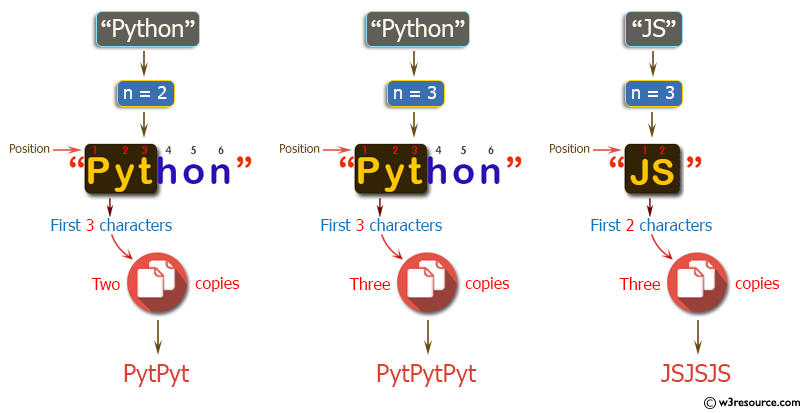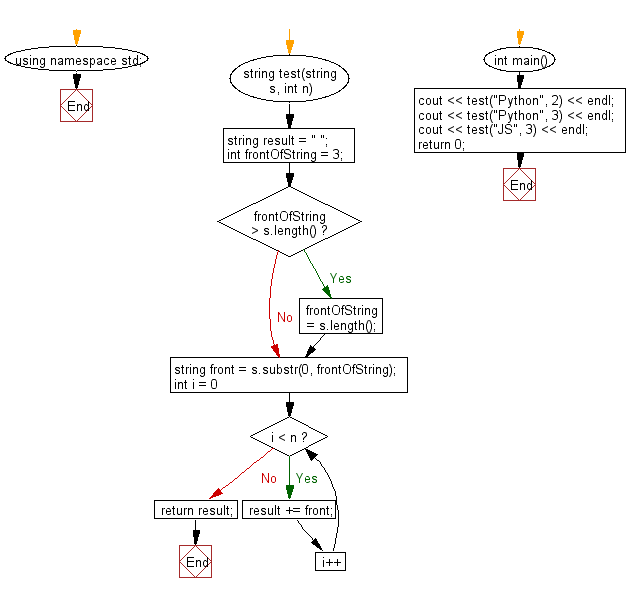﻿ C++ : New string, n copies of first 3 characters of a string# C++ Exercises: Create a new string which is n copies of the first 3 characters of a given string

## C++ Basic Algorithm: Exercise-25 with Solution

Write a C++ program to create a new string which is n (non-negative integer) copies of the first 3 characters of a given string. If the length of the given string is less than 3 then return n copies of the string.

Sample Solution:

C++ Code :

``````#include <iostream>
using namespace std;

string test(string s, int n)
{
string result = " ";
int frontOfString = 3;

if (frontOfString > s.length())
frontOfString = s.length();

string front = s.substr(0, frontOfString);

for (int i = 0; i < n; i++)
{
result += front;
}
return result;
}

int main()
{
cout << test("Python", 2) << endl;
cout << test("Python", 3) << endl;
cout << test("JS", 3) << endl;
return 0;
}
``````

Sample Output:

```PytPyt
PytPytPyt
JSJSJS
```

Pictorial Presentation:Flowchart:C++ Code Editor:

Contribute your code and comments through Disqus.

What is the difficulty level of this exercise?

﻿